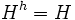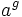# Center of pronormal subgroup is subset-conjugacy-determined in normalizer

CONVENTION WARNING: This article/section uses the right-action convention. The left and right action conventions are equally powerful and statements/reasoning here can be converted to the alternative convention (the main reason being that every group is naturally isomorphic to its opposite group via the inverse map). For more on the action conventions and switching between them, refer to switching between the left and right action conventions.

## Statement

Suppose$G$ is a group and$H$ is a Pronormal subgroup (?). Suppose$A,B$ are subsets of$Z(H)$ (the Center (?) of$H$) and$g \in G$ is such that$A^g = B$. Then, there exists$h \in N_G(H)$ such that, for all$a \in A$,$a^h = a^g$.

(Here,$a^g = g^{-1}ag$ denotes conjugation by$g$, following the right-action convention. The statement and proof remain the same for the left-action convention).

## Definitions used

### Pronormal subgroup

Further information: Pronormal subgroup

A subgroup$H$ of a group$G$ is termed a pronormal subgroup if for any$g \in G$, there exists$x \in \langle H, H^g \rangle$ such that$H^x = H^g$.

## Proof

Given:$H \le G$ is a pronormal subgroup,$A^g = B$ for$A, B \subseteq Z(H)$ and$g \in G$.

To prove: There exists$h \in N_G(H)$ such that$a^g = a^h$ for all$a \in A$.

Proof: Since$A^g = B$, we have$C_G(A)^g = C_G(B)$. Now, since$A \subseteq Z(H)$, we have$H \le C_G(A)$, so$H^g \le C_G(B)$. Also,$B \subseteq Z(H)$, so$H \le C_G(B)$.

Thus,$\langle H, H^g \rangle \le C_G(B)$. By pronormality, there exists$x \in \langle H, H^g \rangle$ such that$H^x = H^g$. In particular, there exists$x \in C_G(B)$ such that$H^x = H^g$.

Now consider$h = gx^{-1}$. We check the two required conditions:

•$h \in N_G(H)$: Clearly,$H^h = H^{gx^{-1}} = (H^g)^{x^{-1}} = (H^x)^{x^{-1}} = H^{xx^{-1}} = H$. So,$H^h = H$, and hence$h \in N_G(H)$.
•$a^g = a^h$ for all$a \in A$: Pick$a \in A$. We have$a^h = a^{gx^{-1}} = (a^g)^{x^{-1}}$. Now,$a^g \in B$ and$x \in C_G(B)$, so$x^{-1}$ fixes$a^g$. Thus,$a^h = a^g$.